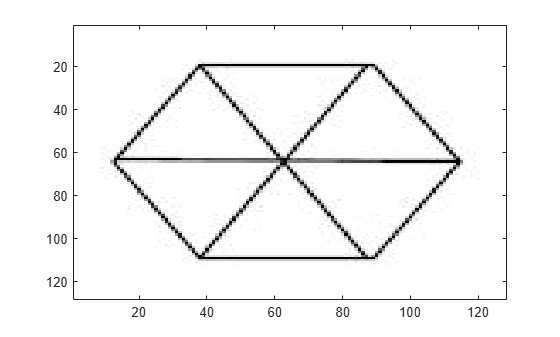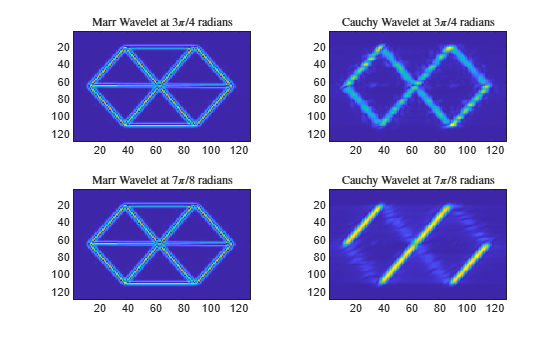# cwtft2

2-D continuous wavelet transform

## Syntax

``cwtstruct = cwtft2(x)``
``cwtstruct = cwtft2(x,'plot')``
``cwtstruct = cwtft2(x,Name,Value)``

## Description

example

````cwtstruct = cwtft2(x)` returns the 2-D continuous wavelet transform (CWT) of the 2-D matrix, `x`. `cwtft2` uses a Fourier transform-based algorithm in which the 2-D Fourier transforms of the input data and analyzing wavelet are multiplied together and inverted.```

example

````cwtstruct = cwtft2(x,'plot')` plots the data and the 2-D CWT.```

example

````cwtstruct = cwtft2(x,Name,Value)` uses additional options specified by one or more Name,Value pair arguments.```

## Examples

collapse all

Shows how an isotropic wavelet does not discern the orientation of features while an anisotropic wavelet does. The example uses the Mexican hat isotropic wavelet and the directional (anisotropic) Cauchy wavelet.

Load and view the hexagon image.

```Im = imread('hexagon.jpg'); imagesc(Im); colormap(jet); ```Obtain the scale-one 2-D CWT with both the Mexican hat and Cauchy wavelets. Specify a vector of angles going from 0 to 15?/8 in ?/8 increments.

```cwtcauchy = cwtft2(Im,'wavelet','cauchy','scales',1,... 'angles',0:pi/8:2*pi-pi/8); cwtmexh = cwtft2(Im,'wavelet','mexh','scales',1,... 'angles',0:pi/8:2*pi-pi/8); ```

Visualize the scale-one 2-D CWT coefficient magnitudes at each angle.

```angz = {'0', 'pi/8', 'pi/4', '3pi/8', 'pi/2', '5pi/8', '3pi/4', ... '7pi/8','pi', '9pi/8', '5pi/4', '11pi/8', '3pi/2', ... '13pi/8' '7pi/4', '15pi/8'}; for angn = 1:length(angz) subplot(211) imagesc(abs(cwtmexh.cfs(:,:,1,1,angn))); title(['Mexican hat at ' angz(angn) 'radians']); subplot(212) imagesc(abs(cwtcauchy.cfs(:,:,1,1,angn))); title(['Cauchy wavelet at ' angz(angn) 'radians']); pause(1); end ```Load an image of a woman, obtain the 2-D CWT using the Morlet wavelet, and plot the CWT coefficients.

```load woman; cwtmorl = cwtft2(X,'scales',1:4,'angles',0:pi/2:3*pi/2,'plot');```Obtain the 2-D CWT of the star image using the default Morlet wavelet, scales `2^(0:5)`, and an angle of 0.

```Im = imread('star.jpg'); cwtout = cwtft2(Im);```

## Input Arguments

collapse all

Input data, specified as a 2-D matrix or 3-D array. If the input data is a 3-D array, the input matrix is a truecolor image.

Example: `X = imread('stars.jpg');`

Data Types: `double` | `uint8`

### Name-Value Arguments

Specify optional comma-separated pairs of `Name,Value` arguments. `Name` is the argument name and `Value` is the corresponding value. `Name` must appear inside quotes. You can specify several name and value pair arguments in any order as `Name1,Value1,...,NameN,ValueN`.

Example: `'wavelet','paul','scales',2^(0:5)` specifies to use the Paul wavelet and a vector of scales.

Angles in radians, specified as a comma-separated pair consisting of `'angles'` and either a scalar or a vector.

Example: `'angles',[0 pi/2 pi]`

Normalization used in the 2-D CWT, specified as a comma-separated pair consisting of `'norm'` and one of these character vectors:

• `'L2'` — The Fourier transform of the analyzing wavelet at a given scale is multiplied by the corresponding scale. `'L2'` is the default normalization.

• `'L1'` — The Fourier transform of the analyzing wavelet is multiplied by 1 at all scales.

• `'L0'` — The Fourier transform of the analyzing wavelet at a given scale is multiplied by the square of the corresponding scale.

Example: `'norm','L1'`

Scales, specified as a comma-separated pair consisting of `'scales'` and either a positive real-valued scalar or a vector of positive real numbers.

Example: `'scales',2^(1:6)`

Analyzing wavelet, specified as a comma-separated pair consisting of `'wavelet'` and a character vector, a string scalar, a structure, or a cell array. `cwtftinfo2` provides a comprehensive list of supported wavelets and associated parameters.

If you specify `'wavelet'` as a structure, the structure must contain two fields:

• `name` — the character vector or string scalar corresponding to a supported wavelet.

• `param` — a cell array with the parameters of the wavelet.

If you specify `'wavelet'` as a cell array, `wav`, the cell array must contain two elements:

• `wav{1}` — the character vector or string scalar corresponding to a supported wavelet.

• `wav{2}` — a cell array with the parameters of the wavelet.

Example: `'wavelet',{'morl',{6,1,1}}`

Example: `'wavelet',struct('name','paul','param',{'p',2})`

## Output Arguments

collapse all

The 2-D CWT, returned as a structure with the following fields:

Analyzing wavelet and parameters, returned as a structure with the following fields:

• `wname` — name

• `param` — parameters

Normalization constants, returned as a M-by-N matrix where M is the number of scales and N is the number of angles.

CWT coefficients, returned as an N-D array. The row and column dimensions of the array equal the row and column dimensions of the input data. The third page of the array is equal to 1 or 3 depending on whether the input data is a grayscale or truecolor image. The fourth page of the array is equal to the number of scales and the fifth page of the array is equal to the number of angles.

Scales for the 2-D CWT, returned as a row vector.

Angles for the 2-D CWT, returned as a row vector.

Mean of the input data, returned as a scalar

### Topics

Introduced in R2013b

## SupportGet trial now